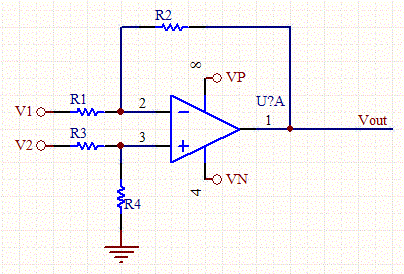# Inverting Op-Amp Resistor Calculator

This calculator determines the bias and feedback resistors for an op-amp, given the gain and desired output bias point. There are many free parameters to the design so enter the value of R1, which will scale the other resistors. Use V1 as the input for the inverting Op-Amp, and V2 as a voltage offset if needed. Set V2 to zero if no offset is required.Op-Amp Circuit Schematic

 Input Parameters (Inverting Op-amp) Vout (V) Gain R1 (KOhms) V1(Input lead) (V) V2 (non-zero only if offset is requred) (V) Vp (V) Vn (V) Outputs R2 (KOhms) R3 (KOhms) R4 (KOhms)